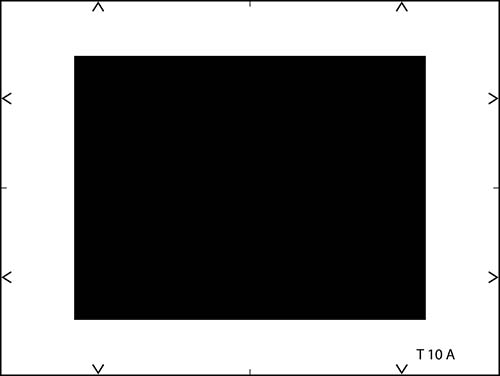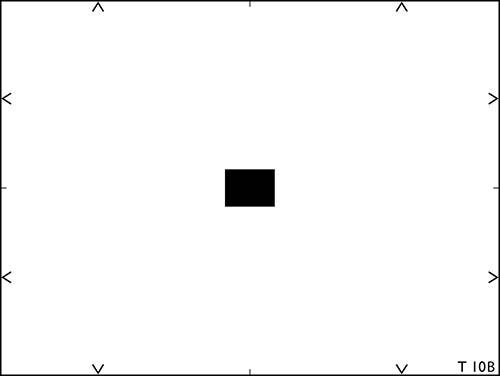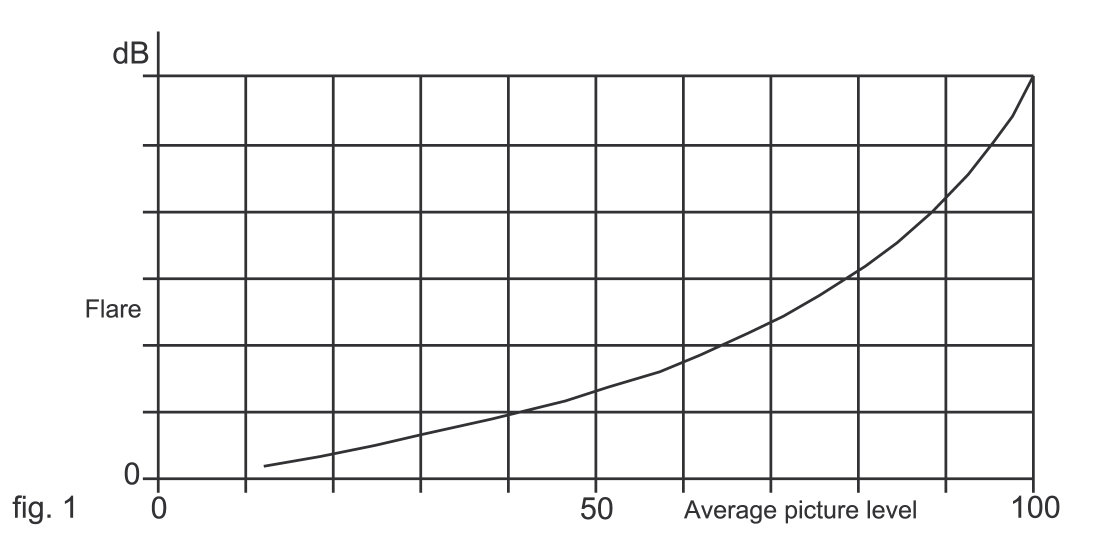# Scattered Light测试卡

• 品牌：ESSER爱莎/Sine Image
型号：T10A、T10B
尺寸：可定制
材料：菲林/高清相纸
比例：4:3
类型：反射式/透射式可选
•### Definition “Flare”

Flare caused by diffusion of light in the optical path (zoom lenses, dichroitic beam splitter) impairs the picture, either by throwing an uniform white veil over the picture or by blurring transistors. It is essential that a distinction is made between these two effects, which implies determining the variations of the black level as compared to its nominal level when the average picture level analyzed varies between 0% to 50% on the one hand, and from 0% to 99% on the other hand, as flare depends on the average picture level as shown in fig.1:### Equipment used

To measure overall flare, the test chart (T10A) has in its center a black rectangle of 4/3 aspect ratio. This rectangle is surrounded by a white zone of equal area, thus giving an average picture level (APL) of 50%

To measure localized flare, the test chart (T10B) has a central black rectangle of 4/3 aspect ratio surrounded by a white zone area such as to result in an average picture level (APL) of 99%.

Oscilloscope

Video amplitude meter

### Measurement conditions

Test chart illumination is adjusted so that a white level of 100% is obtained with an iris aperture of F/4 for 2/3” CCD and F/2.8 for a 1/2” CCD. Measurements are made under different conditions:

Gamma corrector “OFF” or gamma = 1

Flare corrector “ON”

The black level is set at 5% of the nominal level, namely 30mV.

### Measurement procedure

The focal length and aperture of the lens are not changed during the measurements. The camera is centered on the chart with APL of 50%. The change in black level, at the center of the image, is then measured for each of the R, G, B channels. The test chart with an APL of 50% (T10A) is then replaced by the test chart with an APL of 99% (T10B) and the change in black level, at the center of the image, is measured for each of the R, G, B channels. The measurements are repeated for the corners of the image using the second test chart with an APL of 99% (T10B).

### Presentation of results

The results are expressed as percentages of the nominal level of 700mV / 75ohms.

Global Effect

 Gamma = 1 Gamma = 0.45 R G B R G B X % X % X % X % X % X % R – G B – G R – G B – G X % X % X % X %

Localized Effect

 Gamma = 1 Gamma = 0.45 R G B R G B X % X % X % X % X % X % R – G B – G R – G B – G X % X % X % X %

### Standards

Pflichtenheft 8/ 1.1 der öffentlich-rechtlichen Rundfunkanstalten der Bundesrepublik Deutschland “ Richtlinien für die Messung der Pflichtenheftsbedingungen an Videogeräten”

IEC 611461- 2 Video cameras (PAL/SECAM/NTSC) - Methods of Measurement - Part 2: Two- and three-sensor professional cameras, 1997

相关推荐